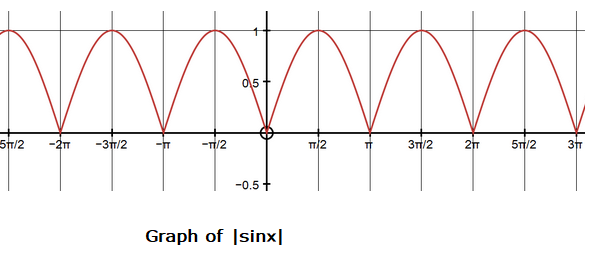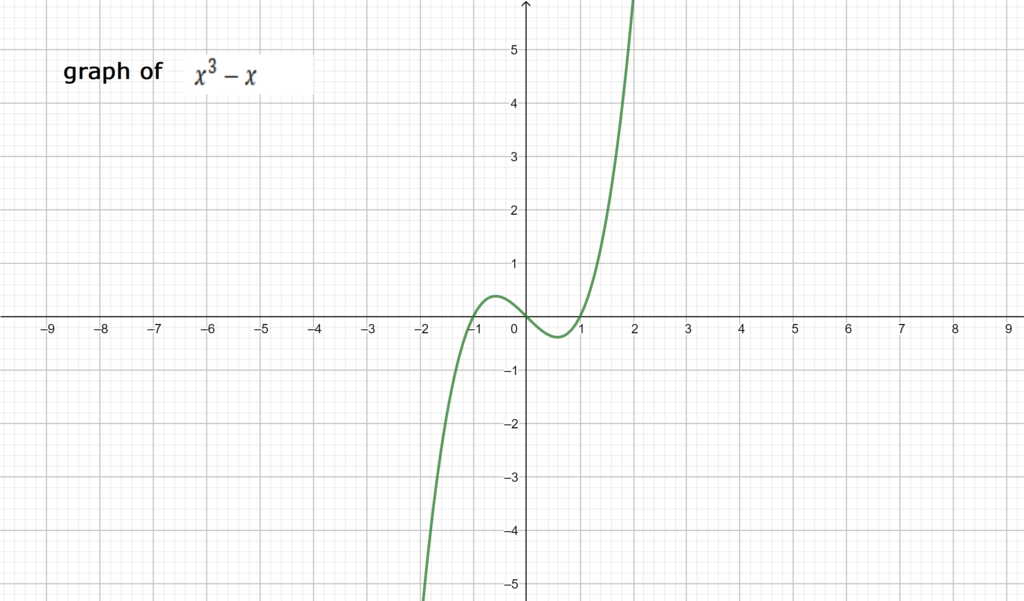October 1, 2023

# Definite integration involving modulus function

Definite  Integration Involving Modulus Function

There is no anti-derivative for a modulus function; however we know it’s definition

Thus we can split up our integral  in two parts. One  part must be completely negative and the another  must be completely positive. This strategy is known as splitting .  To split correctly it is better to  draw the modulus graph so that we can get the correct equation to represent each portion of the modulus graph .

Example 1 Find the value of

.

Sol.  For a periodic function with period , where is any natural number.

Here    whose  period  is .

Hence

=          (  since   )

=   = 9 x 2 =18 .

ThusExample 2.  Evaluate

Sol.  We note that on    and on    and that on .Graph of

So

=

=

=

=

Example 3. Find the value

Sol.  We know that     and   has one common value at .   In the interval  ,    and in   , .  Thus   in   and in .

So

=

=

https://youtu.be/EAYq3ySAy9k#### Bina singh

View all posts by Bina singh →
ssc chsl 2023 tier 1 cut off NIRF Rankings 2023 : Top 10 Engineering colleges in India CBSE Compartment Exam 2023 Application Form SSC CGL 2023 Notification: Important Dates UPSC Recruitment 2023